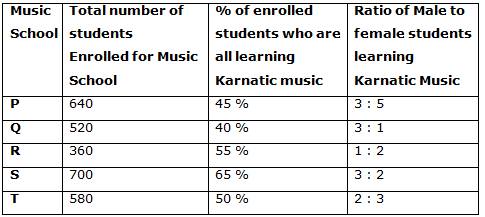# IBPS Clerk Prelims 2018 – Quantitative Aptitude Questions Day-25

Dear Readers, Bank Exam Race for the Year 2018 is already started, To enrich your preparation here we have providing new series of Practice Questions on Quantitative Aptitude – Section. Candidates those who are preparing for IBPS Clerk Prelims 2018 Exams can practice these questions daily and make your preparation effective.

[WpProQuiz 4250]

Directions (Q. 1 – 5): What value should come in place of question mark (?) in the following questions?

1) [(450 ÷ 18)1/2 + (418 ÷ 11)2] = (?)2 + 360

a) 28

b) 24

c) 33

d) 37

e) None of these

2) 42 % of 650 ÷ 21 + 12 % of 225 = ? + 15 % of 200

a) 10

b) 16

c) 25

d) 32

e) None of these

3) 4√x ÷ (12 × 7) + 14 = 23

a) 34225

b) 29584

c) 31684

d) 35721

e) None of these

4) 13 % of 1200 + 162 ÷ 4 + 152 =? % of 400

a) 105

b) 93

c) 84

d) 72

e) None of these

5) 10 % of? – 5310 ÷ 15 – 372 ÷ 4 = 68

a) 4370

b) 3820

c) 5150

d) 6380

e) None of these

Directions (Q. 6 – 10) Study the following information carefully and answer the given questions:

The following table shows the total number of students enrolled in different music schools and the percentage of enrolled students who are learning karnatic music and the ratio of male and female learning karnatic music.6) Find the ratio between the total number of students learning Karnatic music from music school P to that of S?

a) 312: 157

b) 11: 23

c) 288: 455

d) 35: 43

e) None of these

7) Find the total number of male students learning Karnatic music from Music school Q, R and T together?

a) 338

b) 412

c) 436

d) 474

e) None of these

8) Find the difference between the total students learning other than Karnatic music from music school Q to that of T?

a) 44

b) 13

c) 38

d) 22

e) None of these

9) The total students enrolled for music from School R is approximately what percentage of total students enrolled for music from School S?

a) 38 %

b) 51 %

c) 62 %

d) 73 %

e) 84 %

10) The total number of male students enrolled for Karnatic music from School P is approximately what percentage more/less than the total number of female students enrolled for Karnatic music from School T?

a) 38 %

b) 45 %

c) 57 %

d) 22 %

e) 66 %

Direction (1-5) :

[(450 ÷ 18)1/2 + (418 ÷ 11)2] = x2 + 360

251/2 + 382 = x2 + 360

5 + 1444 – 360 = x2

1089 = x2

X = 33

42 % of 650 ÷ 21 + 12 % of 225 = ? + 15 % of 200

[(42/100)*650]/21 + (12/100)*225 – (15/100)*200 = x

13 + 27 – 30 = x

X = 10

4√x ÷ (12 × 7) + 14 = 23

√x/21 = 9

√x = 189

X = 35721

13 % of 1200 + 162 ÷ 4 + 152 = x % of 400

(13/100)*1200 + (16*16)/4 + 152 = (x/100)*400

156 + 64 + 152 = 4x

372 = 4x

X = 93

10 % of x – 5310 ÷ 15 – 372 ÷ 4 = 68

(10/100)*x – (5310/15) – (372/4) = 68

(x/10) = 68 + 354 + 93

(x/10) = 515

X = 5150

Direction (6-10) :

The total number of students learning Karnatic music from music school P

= > 640*(45/100) = 288

The total number of students learning Karnatic music from music school S

= > 700*(65/100) = 455

Required ratio = 288 : 455

The total number of male students learning Karnatic music from Music school Q, R and T together

= > 520*(40/100)*(3/4) + 360*(55/100)*(1/3) + 580*(50/100)*(2/5)

= > 156 + 66 + 116 = 338

The total students learning other than Karnatic music from music school Q

= > 520*(60/100) = 312

The total students learning other than Karnatic music from music school T

= > 580*(50/100) = 290

Required difference = 312 – 290 = 22

The total students enrolled for music from School R = 360

The total students enrolled for music from School S = 700

Required % = (360/700)*100 = 51 %

The total number of male students enrolled for Karnatic music from School P

= > 640*(45/100)*(3/8) = 108

The total number of female students enrolled for Karnatic music from School T

= > 580*(50/100)*(3/5) = 174

Required % = [(174 – 108)/174]*100 = 38 %

Kindly watch this video to know about Top 5 Arithmetic Questions for IBPS Clerk Prelims

Daily Practice Test Schedule | Good Luck

 Topic Daily Publishing Time Daily News Papers & Editorials 8.00 AM Current Affairs Quiz 9.00 AM Current Affairs Quiz (Hindi) 9.30 AM IBPS Clerk Prelims – Reasoning 10.00 AM IBPS Clerk Prelims – Reasoning (Hindi) 10.30 AM IBPS Clerk Prelims – Quantitative Aptitude 11.00 AM IBPS Clerk Prelims – Quantitative Aptitude (Hindi) 11.30 AM Vocabulary (Based on The Hindu) 12.00 PM IBPS PO Prelims – English Language 1.00 PM SSC Practice Questions (Reasoning/Quantitative aptitude) 2.00 PM IBPS PO/Clerk – GK Questions 3.00 PM SSC Practice Questions (English/General Knowledge) 4.00 PM Daily Current Affairs Updates 5.00 PM IBPS PO Mains – Reasoning 6.00 PM IBPS PO Mains – Quantitative Aptitude 7.00 PM IBPS PO Mains – English Language 8.00 PM